# NCERT Solutions class 7 Maths Chapter-10 Exercise 10.5

NCERT Solutions Class-7 Maths chapter-10 line and angle Exercise-10.5 is prepared by academic team of pw all the questions of NCERT text book are solved step by step with proper and detail solutions explaining each and every questions . For More and additional questions of CBSE class 7 maths you can go to class 7 maths sections. NCERT class 7 Maths Solutions is the best way to enhanced your mathematics skill. And pw practice worksheet & question bank will help you a lot .

## NCERT Solutions class 7 Maths Chapter-10 line and angle

### Solutions of Chapter line and angle Exercise-10.5

Question 1:
Construct the right angled ?PQR, where m∠Q = 90°, QR = 8 cm and PR = 10 cm.
A rough sketch of ?PQR is as follows.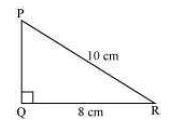The steps of construction are as follows.
(i) Draw a line segment QR of length 8 cm.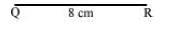(ii) At point Q, draw a ray QX making 90º with QR.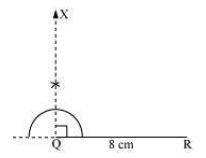(iii) Taking R as centre, draw an arc of 10 cm radius to intersect ray QX at point P.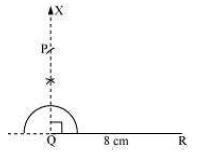(iv) Join P to R. ?PQR is the required right-angled triangle.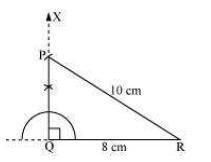Question 2:
Construct a right-angled triangle whose hypotenuse is 6 cm long and one of the legs is 4 cm long.
A right-angled triangle ABC with hypotenuse 6 cm and one of the legs as 4 cm has to be constructed. A rough sketch of ?ABC is as follows.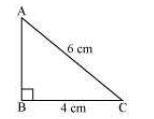The steps of construction are as follows.
(i) Draw a line segment BC of length 4 cm.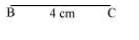(ii) At point B, draw a ray BX making an angle of 90º with BC.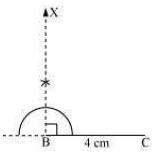(iii) Taking C as centre, draw an arc of 6 cm radius to intersect ray BX at point A.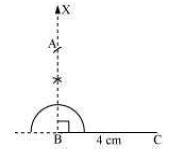(iv) Join A to C to obtain the required ?ABC.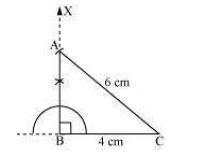Question 3:
Construct an isosceles right-angled triangle ABC, where, m∠ACB = 90° and AC = 6 cm.
In an isosceles triangle, the lengths of any two sides are equal.
Let in ?ABC, AC = BC = 6 cm. A rough sketch of this ?ABC is as follows.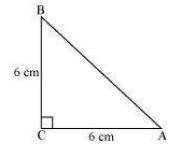The steps of construction are as follows.
(i) Draw a line segment AC of length 6 cm.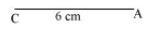(ii) At point C, draw a ray CX making an angle of 90º with AC.(iii) Taking point C as centre, draw an arc of 6 cm radius to intersect CX at point B.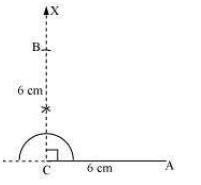(iv) Join A to B to obtain the required ?ABC.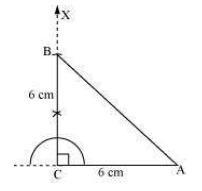NCERT CLASS 7 MATHEMATICS SOLUTIONS